# Beyond Mapping III

Topic 8: Investigating Spatial Dependency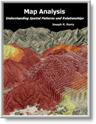Map Analysis book with companion CD-ROM for hands-on exercises

describes the basic non-spatial descriptive statistics
of Spatial Dependency  discusses spatial dependency and illustrates the effects of different spatial arrangements of the same set of data
describes the basic measures of autocorrelation
describes a technique for generating a map of spatial autocorrelation
discuses a procedure to incorporate direction as well as distance for assessing spatial dependency

Note: The processing and figures discussed in this topic were derived using MapCalcTM software.  See www.innovativegis.com to download a free MapCalc Learner version with tutorial materials for classroom and self-learning map analysis concepts and procedures.

______________________________

GIS Data Are Rarely Normal

(GeoWorld, October 1998, pg. 24-26)

Most of us are familiar with the old “bell-curve” for school grades.  You know, with lots of C’s, fewer B’s and D’s, and a truly select set of A’s and F’s.  Its shape is a perfect bell, symmetrical about the center with the tails smoothly falling off toward less frequent conditions.

Although the distribution is familiar and easy to visualize, the normal distribution (bell-shaped) isn’t as normal (typical) as you might think.  For example, Newsweek recently noted that the average grade at a major ivy-league university isn’t a solid C with a few A’s and F’s sprinkled about as you might imagine, but an A- with a lot of A’s trailing off to lesser amounts of B’s, C’s and (heaven forbid) the rare D’s and F’s.

The frequency distributions of mapped data also tend toward the ab-normal (formally termed asymmetrical).  For example, consider the elevation data shown in figure 1.  The contour map and 3-D surface on the left depict the geographic distribution of the data.  Note the distinct pattern of the terrain with higher elevations in the northeast and lower ones along the western portion.  As is normally the case with mapped data, the elevation values are neither uniformly nor randomly distributed in  geographic space.  The unique pattern is the result complex physical processes driven by a host of factors—not spurious, arbitrary, constant or even “normal” events.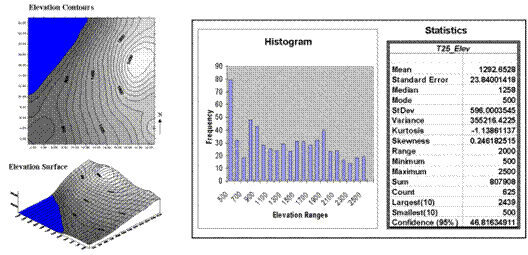Figure 1.  Mapped data are characterized by their geographic distribution (maps on the left) and their numeric distribution (histogram and statistics on the right).

Now turn your attention to the numeric distribution of the data depicted in the right side of the figure.  The data view was generated by simply transferring the gridded elevation values to Excel, then applying the Histogram and Descriptive Statistics options of the Data Analysis add-in tools. The mechanics used to plot the histogram and generate the statistics were a piece-of-cake, but the real challenge is to make some sense of it all.  Note that the data aren’t distributed as a normal bell-curve, but appear flattened and slightly shifted to the left.  The tall spike at the lowest elevation range (500-600 feet) is due to the lake in the northwest corner.  If the lake was drained (or its bathometry considered) some of the spike’s values would be assigned smaller elevations and the distribution would broaden and flatten even more.

If the terrain contained a plateau or mesa instead of the smooth hill in the northeast, there would be a spike at the high end of the histogram.  What do you think the histogram would look like if the area contained several chimney-rocks or “whoodoos” scattered about a flat plain?  Or if the area were centered on an escarpment?

The mental exercise linking geographic space with data space is a good one, and some general points ought to be noted.  First, there isn’t a fixed relationship between the two views of the data’s distribution (geographic and data).  A myriad of geographic patterns can result in the same histogram.  That’s because spatial data contains additional information—where, as well as what—and the same data summary of the “what’s” can reflect a multitude of spatial arrangements (“where’s).

But is the reverse true?  Can a given geographic arrangement result in different data views?  Nope, and it’s this relationship that catapults mapping and geo-query into the arena of mapped data analysis.  Traditional analysis techniques assume a functional form for the frequency distribution (histogram shape), with the standard normal (bell-shaped) being the most prevalent.  Last June’s column described the basic descriptive statistics Excel’s summary table— maximum, minimum, range, mode, median, mean (average), variance, standard deviation and an additional one termed coefficient of variation.  The discussion described how these statistics portray the central tendency (typical condition) of a data set.  In effect, they reduce the complexity of a large number of measurements to just a handful of numbers and provide a foothold for further analysis.

A brief discussion of the additional indices in Excel’s table is warranted.  The sum and the count should be obvious—the total of all the measurements (sum= 807,908 “total” feet above sea level doesn’t mean much in this context) and the number of measurements (count= 625 data values indicates a fairly big data set as traditional statistics go, but fairly small for spatial statistics).  The largest/smallest statistic in the table identifies the average of a user-specified number of values (10 in this case) at the extreme ends of the data set.  It is interesting to note that the average of the 10 smallest elevation values (500) is the same as the minimum value, while the average of the 10 largest values (2439) is well below the maximum value of 2500.

The standard error calculates the average difference between the individual data values and the mean (StdError= sum [[x-mean]**2] / [n*[n-1]]).  If the average deviation is fairly small, then the mean is fairly close to each of the sample measurements.  The standard error for the elevation data is 23.84001418 (whoa Excel, way too many decimals—nothing in statistics is that precise).  The statistic means that the mean is on the average (got that?) about 24 feet above or below the 625 individual elevation values comprising the map.  Useful information, but often the attention of most GIS applications is focused on the areas of “unusually” high or low areas (the outliers), not how well the average “fits” the entire data set.

The confidence level is a range on either side of a sample mean that you are fairly sure contains the population (true) average. For example, if you have some data on mail order delivery times, you can determine, with a particular level of confidence (usually 95%), the earliest and latest a product will likely arrive.

The elevation data’s confidence value of 46.81634911 suggests that we can be fairly sure that the “true” average elevation is between 1245 and 1340.  But this has a couple of important assumptions—that the data represents a good sample and that the normal curve is a good representation of the actual distribution.

But what if the distribution isn’t normal?  What if it is just a little ab-normal?  What if it is a lot?  That’s the stuff of doctoral theses, but there are some general considerations that ought to be noted.  First, there are some important statistics that provide insight into how normal a data set is.  Skewness tells us if the data is lop-sided.  Formally speaking, it “characterizes the degree of asymmetry of a distribution around its mean.” Positive skewness indicates a distribution shifted to left, while negative skewness indicates a shift to the right and 0 skewness is indicates perfectly symmetrical data.  The larger the value, the more pronounced is the lop-sided shift.  In the elevation data, a skewness value of .246182515 indicates a slight shift to the right.

Another measure of ab-normality is termed kurtosis.  It characterizes the relative “peakedness or flatness” of a distribution compared with the “ideal” bell-shaped distribution. A positive kurtosis indicates a relatively peaked distribution, while a negative kurtosis indicates a relatively flat one and 0 is just the right amount (sounds like Goldilock’s “papa, mamma and baby bear” sizing of distribution shape).  Its magnitude reports the degree of distortion from a perfect bell-shape.  The –1.13861137 kurtosis value for the elevation data denotes a substantial flattening.

All in all, the skewness and kurtosis values don’t bode well for the elevation data being normally distributed.  In fact, a lot of spatial data isn’t very normal…some might say most.  So what do you do?  Throw away the Excel-type descriptive statistics?  Punt on statistical analysis and simply generate really cool graphics for visceral visions of the relationships?  Do you blindly go ahead and impose assumptions of normalcy just to force-fit normal analysis procedures?  Good questions, but they will have to wait for the next section’s discussions.

(GeoWorld, November 1998, pg. 28-30)

The previous section investigated the numerical character of a gridded elevation surface.  Keystone to the discussion was the degree of “normality” exhibited in the data as measured by commonly used descriptive statisticsmin, max, range, median, mode, mean (or average), variance, standard deviation, standard error, confidence level, skewness and kurtosis.  All in all, it appeared that the elevation data didn’t fit the old “bell-shaped” curve very well.

So, how useful is “normal” statistics in GIS applications?  That’s an interesting debate set off by a letter to the editor (GIS World, Vol. 11, No. 9), with several individuals contributing their thoughts to the “Column Supplements” page at www.innovativegis.com/basis.  If you’re a techy-type and wired to the net you might want to check out the extended discussion.

At the risk of overstepping my bounds of expertise, let me suggest that using the average to represent the central tendency of a data set is usually OK.  However, when the data isn’t normally distributed the average might not be a good estimator of the “typical” condition.  Similarly, the standard deviation can be an ineffective measure of dispersion for ab-normally distributed data… it all depends

So, what’s a GIS’er to do, short of getting an advanced degree in statistics or abducting a statistician?  The correct response is to enter the murky realm of non-parametric statistics.  The easy response is to forget the last several columns and blissfully apply the average and standard deviation to all of the data falling within a polygon.  Yet another response is to use the “poor man’s” answer to asymmetric data— use the median to represent the “typical” condition and the quartile range to estimate the data’s dispersion (the quartile range corresponds to the middle 50% of the frequency distribution).  Suggesting such a “seat-of-the-pants” statistical procedure should provide the last wack to my extended neck and set-off another round of discussions in the Column Supplements.

Even more disturbing, however, is the realization that while descriptive statistics might provide insight into the numerical distribution of the data, they provide no information what-so-ever into the spatial distribution of the data.  As noted last month, all sorts of terrain configurations can produce exactly the same set of descriptive statistics.  That’s because traditional measures are breed to ignore geographic patterns— in fact spatial independence is an underlying assumption.

So how can one tell if there is spatial dependency locked inside a data set?  You know, Tobler’s first law of geography that “all things are related but nearby things are more related than distant things.”  Let’s use Excel* and some common sense to investigate this keystone concept and the approach used in deriving a descriptive statistics that tracks spatial dependency.

The left side of the figure 1 identifies sixteen sample points in a 25 column by 25 row analysis grid (origin at 1, 1 in the upper left, northwest corner).  The positioning of the samples are depicted in the two 3-D plots.  Note that the sample positions are the same (horizontal axes), only the measurements at each location vary (vertical axis).  The plot on the left depicts sample values that form a plane constantly increasing from the southwest to the northeast.  The plot on the right depicts a jumbled arrangement of the same measurement values.

Figure 1.  The spatial dependency in a data set compares the “typical” and “nearest neighbor” differences— if the nearest neighbor differences are less than the typical differences, then "nearby things are more similar than distant things."

The first column (labeled Value) in the Tilted and Jumbled worksheets confirm that the traditional descriptive statistics are identical— derived from same values, just in different positions.  The second column calculates the difference between each value and the average of the entire set of samples.  The sign of the difference indicates whether the value is above or below the average, or typical value.  The third column (labeled Unsigned) identifies the magnitude of the difference by taking its absolute value— |Value – Average|.  The average of all the unsigned differences summarizes the “typical” difference.  The relatively large figure of 5.50 for both the Tilted and Jumbled data sets establishes that the individual samples aren’t very similar overall.

The next three columns in both worksheets provide insight into the spatial dependency in the two data sets by evaluating Tobler’s first law.  The NN_Value column identifies the value for the nearest neighboring (closest) sample.  It is determined by solving for the distance from each sample location to all of the others using the Pythagorean theorem (c2= a2 + b2), then assigning the measurement value of the closest sample.  The final two columns calculate the unsigned difference between the value at a location and its nearest neighboring value, then compute the unsigned difference— |Value – NN_Value|.   Note that the Tilted data’s nearest neighbor difference (4.38) is considerably less than that for the Jumbled data (9.00).

Now the stage is set.  If the nearest neighbor differences are less than the typical differences, then “…nearby things are more related than distant things.”  A simple Spatial Dependency Measure is calculated as the ratio of the two differences.  If the measure is 1.0, then minimal spatial dependency exists.  As the measure gets smaller, increased positive spatial dependency is indicated; as it gets larger, increased negative spatial dependency is indicated (nearby things are less similar than distant things).

OK, so what if the basic set of descriptive statistics can be extended to include a measure of spatial dependency?  What does it tell you?  How can you use it?  Its basic interpretation is to what degree the data forms a discernable spatial pattern.  If spatial dependency is minimal or negative there is little chance that geographic space can be used to explain the variation in the data.  In these conditions, assigning the average (or median) to an entire polygon is warranted.  On the other hand, if strong positive spatial dependency is indicated, you might consider subdividing the polygon into more homogenous parcels to better “map the variation” locked in a data set.  Or better yet, treat the area as a continuous surface (gridded data).  But further discussion of refinements in calculating and interpreting spatial dependency must be postponed until next time.

_____________________________

*Note:  the Excel worksheets supporting the discussions of the Tilted and Jumbled data sets (as well as a Blocked and a Random pattern) can be downloaded from the “Column Supplements” page at www.innovativegis.com/basis.

(GeoWorld, December 1998, pg. 28)

Recall last month's discussion of "nearest neighbor" spatial dependency to test the assertion that "nearby things are more related than distant things."  The procedure was simple—calculate the difference between each sample value and its closest neighbor (|Value - NN_Value|), then compare them to the differences based on the typical condition (|Value - Average|).  If the Nearest Neighbor and Average differences are about the same, little spatial dependency exists.  If the nearby differences are substantially smaller than the typical differences, then strong positive spatial dependency is indicated and it is safe to assume that nearby things are more related.

But just how are they related?  And just how far is "nearby?"  To answer these questions the procedure needs to be expanded to include the differences at the various distances separating the samples.  As with the previous discussions, Excel can be used to investigate these relationships.*  The plot on the left side of Figure 1, identifies the positioning and sample values for the Tilted Plane data set described last month.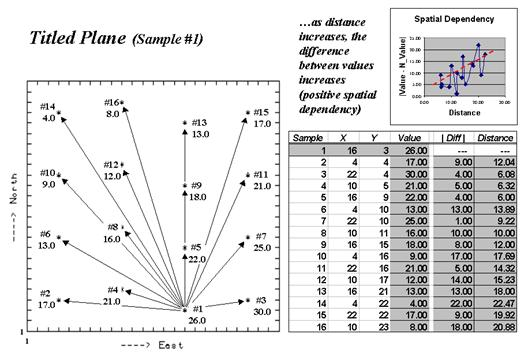Figure 1.   Spatial dependency as a function of distance for sample point #1.

The arrows emanating from sample #1 shows its 15 paired values.  The table on the right summarizes the unsigned differences (|Diff |) and distances (Distance) for each pair.  Note that the "nearby" differences (e.g., #3= 4.0, #4= 5.0 and #5= 4.0) tend to be much smaller than the "distant" differences (e.g., #10= 17.0, #14= 22.0, and #16= 18.0).  The graph in the upper right portion of the figure plots the relationship of sample differences versus increasing distances.  The dotted line shows a trend of increasing differences (a.k.a. dissimilarity) with increasing distances.

Now imagine calculating the differences for all the sample pairs in the data set—the 16 sample points combine for 120 sample pairs—(N*(N-1)/2)= (16*15)/2= 120).  Admittedly, these calculations bring humans to their knees, but it's just a microsecond or so for a computer.  The result is a table containing the |Diff | and Distance values for all of the sample pairs.

The extended table embodies a lot of information for assessing spatial dependency.  The first step is to divide the samples into two groups— close and distant pairs.  For consistency across data sets, let's define the "breakpoint" as a proportion of the maximum distance (Dmax) between sample pairs.  Figure 2 shows the results of applying a dozen breakpoints to divide the data set into "nearby" and "distant" sample sets.  The first row in the table identifies very close neighbors (.005Dmax= 6.10) and calculates the average nearby differences (|Avg_Nearby|) as 4.00.  The remaining rows in the table track the differences for increasing distances defining nearby samples.  Note that as neighborhood size increases, the average difference between sample values increases.  For this data set, the greatest difference occurs for the neighborhood that captures all of the data (1.00Dmax= 25.46 with an average difference of 8.19).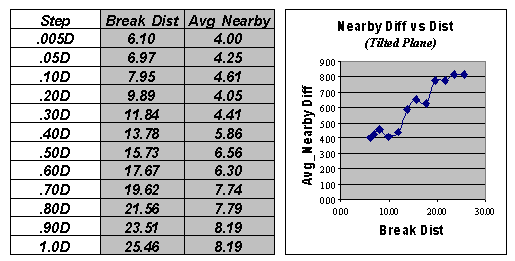Figure 2.   Average “nearby” differences for increasing breakpoint distances used to define neighboring samples.

The techy-types among us will note that the plot of “Nearby Diff vs. Dist” in Figure 28-4 is similar to that of a variogram.  Both assess the difference among sample values as a function of distance.  However, the variogram tracks the difference at discrete distances, while the “Nearby Diff vs Dist” plot considers all of the samples within increasingly larger neighborhoods.

This difference in approach allows us to directly assess the essence of spatial dependency—whether “nearby things are more related than distant things.”  A distance-based spatial dependency measure (SD_D) can be calculated as— SD_D = [|Avg_Distant| - |Avg_Nearby|] / |Avg_Distant|.

The effect of this processing is like passing a donut over the data.  When centered on a sample location, the “hole” identifies nearby samples, while the “dough” determines distant ones.  The “hole” gets progressively larger with increasing breakpoint distances.  If, at a particular step, the nearby samples are more related (smaller |Avg_Nearby| differences) than the distant set of samples (larger |Avg_Distant| differences), positive spatial dependency is indicated.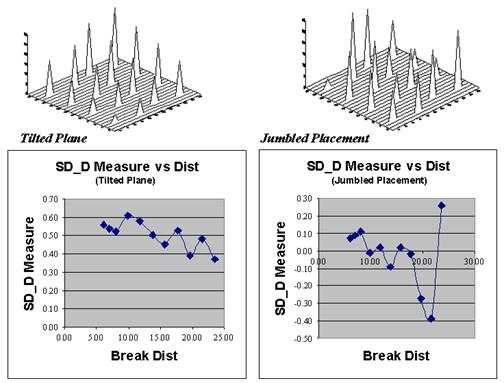Figure 3.  Comparing spatial dependency by directly assessing differences of a sample’s value to those within nearby and distant sets.

Now let’s put the SD_D measure to use.  Figure 28-5 plots the measure for the Tilted Plane (TP with constantly increasing values) and Jumbled Placement (JP with a jumbled arrangement of the same values) sample sets used last month.  First notice that the measures for TP are positive for all breakpoint distances (nearby things are always more related), whereas they bounce around zero for the JP pattern.  Next, notice the magnitudes of the measures— fairly large for TP (big differences between nearby and distant similarities); fairly small for JP.  Finally, notice the trend in the plots—downward for TP (declining advantage for nearby neighbors); flat, or unpredictable for JP.

So what does all this tell us?  If the sign, magnitude and trend of the SD_D measures are like TP’s, then positive spatial dependency is indicated and the data conforms to the underlying assumption of most spatial interpolation techniques.  If the data is more like JP, then “interpolator beware.”

_____________________________

*Note: the Excel worksheets supporting the discussion of the Tilted and Jumbled data sets (as well as a Blocked and Random pattern) can be downloaded from the “Column Supplements” page at www.innovativegis.com/basis.

(GeoWorld, January 1999, pg. 26-27)

The past three columns have focused on the important geographical concept of spatial dependency— that nearby things are more related than distant things.   The discussion to date has involved sets of discrete sample points taken from a variety of geographic distributions.  Several techniques were described to generate indices tracking the degree of spatial dependency in point sampled data.

Now let’s turn our attention to continuously mapped data, such as satellite imagery, soil electric conductivity, crop yield or product sales surfaces.  In these instances, a grid data structure is used and a value is assigned to each cell based on the condition or character at that location.  The result is a set of data that continuously describes a mapped variable.  These data are radically different from point sampled data as they fully capture the spatial relationships throughout an entire area.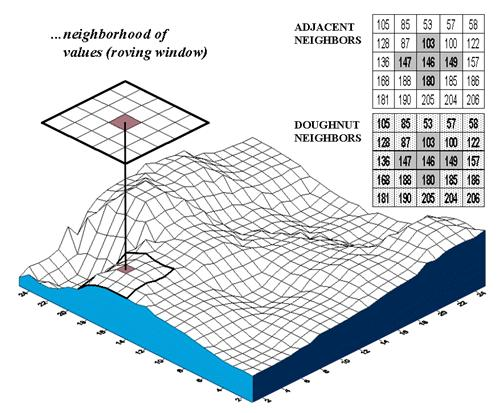Figure 1.  Spatial dependency in continuously mapped data involves summarizing the data values within a “roving window” that is moved throughout a map.

The analysis techniques for spatial dependency in these data involve moving a “roving window” throughout the data grid.  As depicted in figure 1, an instantaneous moment in the processing establishes a set of neighboring cells about a map location.  The map values for the center cell and its neighbors are retrieved from storage and depending on the technique, the values are summarized.  The window is shifted so it centers over the next cell and the process is repeated until all map locations have been evaluated.  Various methods are used to deal with incomplete windows occurring along map edges and areas of missing data.

The configuration of the window and the summary technique is what differentiates the various spatial dependency measures.  All of them, however, involve assessing differences between map values and their relative geographic positions.  In the context of the data grid, if two cells are close together and have similar values they are considered spatially related; if their values are different, they are considered unrelated, or even negatively related.

Geary’s C and Moran’s I, introduced in the 1950’s, are the most frequently used measures for determining spatial autocorrelation in mapped data.  Although the equations are a bit intimidating—

Geary’s C = [(n –1) SUM wij (xixj)2] / [(2 SUM wij) SUM (xi – m)2]

Moran’s I = [n SUM wij (xi – m) (xj – m)] / [(SUM wij) SUM (xi – m)2]

where, n = number of cells in the grid
m = the mean of the values in the grid
xi = value of cell in group i and xj = value of cell in group j
wij = a switch set to 1 if the cells are adjacent; 0 if not adjacent (diagonal)

—the underlying concept is fairly simple.

For example, Geary’s C simply compares the squared differences in values between the center cell and its adjacent neighbors (numerator tracking “xixj”) to the overall difference based on the mean of all the values (denominator tracking “xi – m”).  If the adjacent differences are less, then things are positively related (similar, clustered).  If they are more, then things are negatively related (dissimilar, checkerboard).  And if the adjacent differences are about the same, then things are unrelated (independent, random).  Moran’s I is a similar measure, but relates the product of the adjacent differences to the overall difference.

Now let’s do some numbers.  An adjacent neighborhood consists of the four contiguous cells about a center cell, as highlighted in the upper right inset of figure 1.  Given that the mean for all of the values across the map is 170, the essence for this piece of Geary’s puzzle is

C = [(146-147)2 + (146-103) 2 + (146-149) 2 + (146-180) 2] / [4 * (146-170) 2]
= [ 1 + 1849 + 9 + 1156 ] / [ 4 * 576 ]  = 3015 /2304 = 1.309

Since the Geary’s C ratio is just a bit more than 1.0, a slightly uncorrelated spatial dependency is indicated for this location.  As the window completes its pass over all of the other cells, it keeps a running sum of the numerator and denominator terms at each location.  The final step applies some aggregation adjustments (the “eye of newt” parts of the nasty equation) to calculate a single measure encapsulating spatial autocorrelation over the whole map— a Geary’s C of 0.060 and a Moran’s I of 0.943 for the map surface shown in figure 1.  Both measures report strong positive autocorrelation for the mapped data.  The general interpretation of the C and I statistics can be summarized as follows.

 0 < C < 1 Strong positive autocorrelation I  > 0 C > 1 Strong Negative autocorrelation I < 0 C = 1 Random distribution of values I = 0

In the tradition of good science, let me suggest a new, related measure— Berry’s ID.  This Intuitive Dependency (ID) measure simply assigns the calculated ratio from Geary’s formula to each map location.  The result is a map indicating the spatial dependency for each location (pieces of the puzzle), instead of a single value summarizing the entire map.  In the example, 1.309 is assigned to the center location in the figure.  However a value of 0.351 is assigned to the cell directly above it and 4.675 is assigned to the cell directly below it …you do the math.

Although this new measure might be intuitive—adjacent differences (nearby things) versus overall difference (distant things)—it’s much too ugly for statistical canonization.  First, the values are too volatile and aren’t constrained to an easily interpreted range.  More importantly, the measure doesn’t directly address “localized spatial autocorrelation” because the nearby differences are compared to distant differences represented as the map mean.

That’s where the doughnut neighborhood comes in.  The roving window is divided into two sets of data—the adjacent values (inside ring of nearby things) and the doughnut values (outside ring of distant things).  One could calculate the mean for the doughnut values and substitute it for Geary’s C’s denominator.  But since there’s just a few numbers in the outer ring, why not use the actual variation between the center and each doughnut value?  That directly assesses whether nearby things are more related than distant things for each map neighborhood.  A user can redefine “distant things” simply by changing the size of the window.  In fact, if you recall last month’s article, a series of window sizes could be evaluated and differences between the maps at various “doughnut radii” could provide information about the geographic sensitivity of spatial dependency throughout the mapped area (sort of a mapped variogram).

But let’s take the approach one step further for a new measure we might call Berry IT (yep, you got it… "bury it" for tracking the Intimidating Territorial autocorrelation).  Such a measure is reserved for the statistically adept as it performs an F-test for significant difference between the adjacent and doughnut data groups for each neighborhood.  Check out this month’s Column Supplement for more info and an Excel worksheet applying several concepts for mapping spatial
dependency.

Use Polar Variograms to Assess Distance and Direction Dependencies

(GeoWorld, September 2001, pg. 24)

The previous columns have investigated spatial dependency—the assumption that “nearby things are more related than distant things.”  This autocorrelation forms the basic concept behind spatial interpolation and the ability to generate maps from point sampled data.  If there is a lot of spatial autocorrelation in a set of samples, expect a good map; if not, expect a map of pure, dense gibberish.

An index of spatial autocorrelation compares the differences between nearby sample pairs with those from the average of the entire data set.  One would expect a sample point to be more like its neighbor than it is to the overall average.  The larger the disparity between the nearby and average figures the greater the spatial dependency and the likelihood of a good interpolated map.

A variogram plot takes the investigation a bit farther by relating the similarity among samples to the array of distances between them.  Figure 1 outlines the mechanics and important aspects of the relationship.  The distance between a pair of points is calculated by the Pythagorean theorem and  plotted along the X-axis.  A normalized difference between sample values (termed semi-variance) is calculated and plotted along the Y-axis.  Each point-pair is plotted and the pattern of the points analyzed.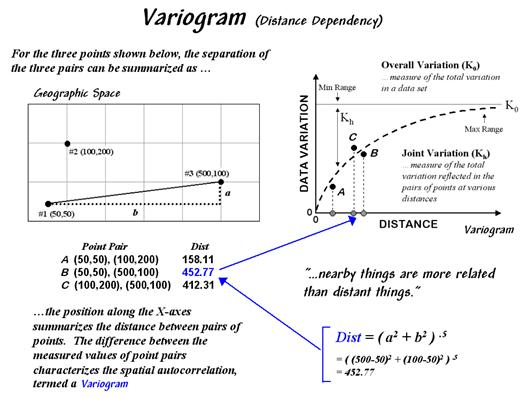Figure 1.  A variogram relates the difference between sample values and their distance.

Spatial autocorrelation exists if the differences between sample values systematically increase as the distances between sample points becomes larger.  The shape and consistency of the pattern of points in the plot characterize the degree of similarity.  In the figure, an idealized upward curve is indicated.  If the remaining point-pairs continue to be tightly clustered about the curve considerable spatial autocorrelation is indicated.  If they are scattered throughout the plot without forming a recognizable pattern, minimal autocorrelation is present.

The “goodness of fit” of the points to the curve serves as an index of the spatial dependency—a good fit indicates strong spatial autocorrelation.  The curve itself provides relative weights for the samples surrounding a location as it is interpolated—the weights are calculated from the equation of the curve.

A polar variogram takes the concept a step further by considering directional bias as well as distance.  In addition to calculating distance, the direction between point-pairs is determined using the “opposite-over-adjacent (tangent)” geometry rule.  A polar plot of the results is constructed with rings of increasing distance divided into sectors of different angular relationships (figure 2).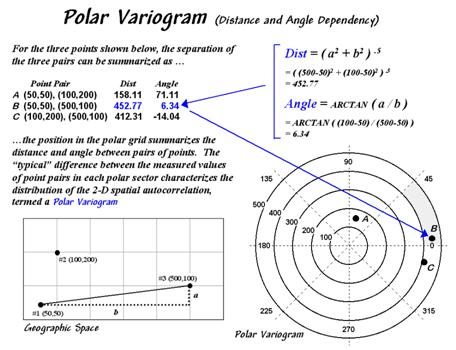Figure 2.  A polar variogram relates the difference between sample values to both distance and direction.

Each point-pair plots within one of the sectors (shaded portion in figure 2).  The difference between the sample values within each sector forms a third axis analogous to the “data variation” (Y-axis) in a simple variogram.

The relative differences for the sectors serve as the weights for interpolation.  During interpolation, the distance and angle for a location to its surrounding sample points are computed and the weights for the corresponding sectors are used.  If there is a directional bias in the data, the weights along that axis will be larger and the matching sample points in that direction will receive more importance.

The shape and pattern of the polar variogram surface characterizes the distance and directional dependencies in a set of data—the X and Y axes depict distance and direction between points while the Z-axis depicts the differences between sample values.

An idealized surface is lowest at the center and progressively increases.  If the shape is a perfect bowl, there is no directional bias.  However, as ridges and valleys are formed directional dependencies are indicated.  Like a simple variogram, a polar variogram provides a graphical representation of spatial dependency in a data set—it just adds direction to the mix.
_____________________________